## How to Calculate and Solve for the Area, Length and Height of a Trapezium | The Calculator Encyclopedia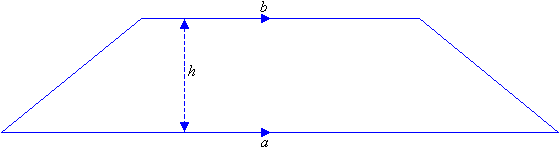The image above is a Trapezium

To compute the area of a trapezium, three essential parameter is needed and they are the length of the top side (a), length of the bottom side (b) and the height of the trapezium (h).

The formula for calculating the area of a trapezium is;

A = 0.5[a + b]h

Where;
A = Area of a trapezium
a = length of the top side of the trapezium
b = length of the bottom side of the trapezium
h = height of the trapezium

Let’s solve an example;
Find the area of a trapezium where length of top side (a) is 7 cm, length of bottom side (b) is 12 cm and height of trapezium (h) is 10 cm.

This implies that;
a = Length of top side of the trapezium = 7 cm
b = Length of bottom side of the trapezium = 12 cm
h = Height of the trapezium = 10 cm

A = 0.5[a + b]h
A = 0.5[7 + 12]10
A = 0.510
A = 95

Therefore, the area of a trapezium is 95 cm2

How to Calculate the Height of a Trapezium when the Area, Length of top side and Length of bottom side of the Trapezium is given.

The formula is h = A / 0.5(a + b)

Where;
A = Area of the trapezium
a = Length of the top side of the trapezium
b = Length of the bottom side of the trapezium

Let’s solve for an example;
Given that the length of top side (a) is 10 cm, length of bottom side (b) is 14 cm and the area of the trapezium is 20 cm2 Find the height of the trapezium?

This implies that;

A = Area of the trapezium = 20 cm2
a = Length of top side of the trapezium = 10 cm
b = Length of bottom side of the trapezium = 14 cm

h = A / 0.5(a + b)
h = 20 / 0.5(10 + 14)
h = 20 / 0.5(24)
h = 20 / 12
h = 1.667

Therefore, the height of the trapezium is 1.667 cm.

## How to Calculate and Solve for the Perimeter and Length of a Square | The Calculator Encyclopedia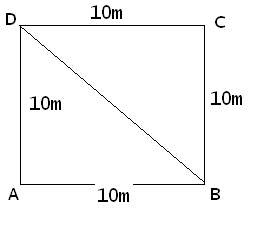The image above represents a square.
To compute the perimeter of a square requires one essential parameter which is the length of one side of a square.

The formula for computing the perimeter of a square is:

P = 4 x l

Where:
P = Perimeter of a Square
l = Length of one side of a Square

Let’s solve an example
Find the perimeter of a square which has a length of 10 m.

This implies that:
l = length of one side of a square = 10

P = 4 x l
P = 4 x 10
P = 40

Therefore, the perimeter of the square is 40 m.

Calculating the Length of a Square when Perimeter is Given

The formula is l = p / 4

Where;
P = Perimeter of a square
l = Length of a square

Let’s solve an example:
Find the length of a square where the perimeter of a square is 100 m.

This implies that;
P = Perimeter of a square = 100 m

l = p / 4
l = 100 / 4
l = 25

Therefore, the length of the square is 25 m.

## How to Calculate and Solve for the Area, Width and Length of a Rectangle | The Calculator Encyclopedia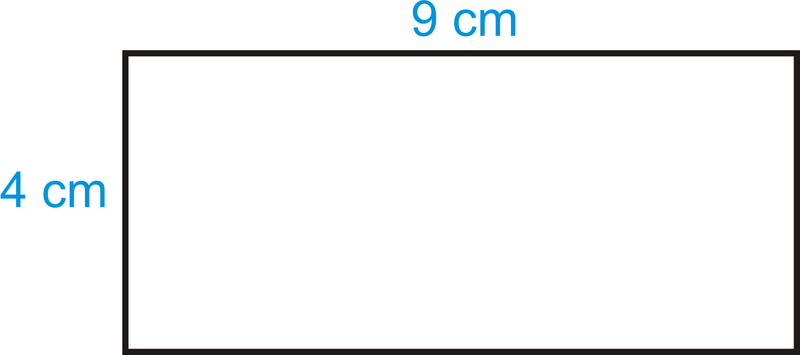The image above is a rectangle with a length of 9 cm and a width of 4 cm.
To compute the area of a rectangle requires two essential parameters which are the length of the rectangle and the width of the rectangle.

The formula for calculating the area of a rectangle is:

A = lw

Where:

A = Area of a Rectangle
l = Length of a Rectangle
w = Width of a Rectangle

Let’s solve an example
Find the area of a rectangle where the length of the rectangle is 9 cm and the width of the rectangle is 4 cm.

This implies that:
l = Length of the Rectangle = 9 cm
w = Width of the Rectangle = 4 cm

A = lw
A = (9)(4)
A = 36

Therefore, the area of the rectangle is 36 cm2.

How to Calculate the Length of a Rectangle when the Area and Width of the Rectangle is Given

The formula for calculating the length of the rectangle is:

l = A / w

Where:

l = Length of the Rectangle
A = Area of the Rectangle
w = Width of the Rectangle

Let’s solve an example:
Find the length of a rectangle where the area of the rectangle is 45 cm2 and the width of the rectangle is 9 cm.

This implies that:
A = Area of the Rectangle = 45 cm2
w = Width of the Rectangle = 9 cm

l = A / w
l = 45 / 9
l = 5

Therefore, the length of the rectangle is 5 cm.

How to Calculate the Width of a Rectangle when the Area and Length of the Rectangle is Given

The formula for calculating the width of the rectangle is:

w = A / l

Where:

w = Width of the Rectangle
A = Area of the Rectangle
l = Length of the Rectangle

## How to Calculate and Solve for the Area and Length of a Square | The Calculator Encyclopedia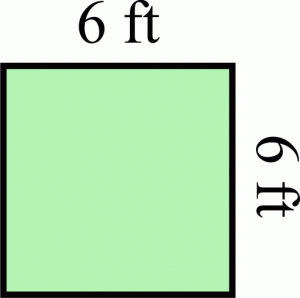The image above represents a square.
To compute the area of a square requires one essential parameter which is the length of one side of a square.

The formula for computing the area of a square is:

A = l2

Where:
A = Area of a Square
l = Length of one side of a Square

Let’s solve an example
Find the area of a square which has a length of 6 ft.

This implies that:
l = length of one side of a square = 6

A = l2
A = 62
A = 6 x 6
A = 36

Therefore, the area of the square is 36 ft2.

Calculating the Length of a Square when Area is Given

The formula is l = √[A]

Where;
A = Area of a square
l = Length of a square

Let’s solve an example:
Find the length of a square where the area of a square is 81 ft2.

This implies that;
A = Area of a square = 81 ft2

l = √[A]
l = √
l = 9

Therefore, the length of the square is 9 ft.

## How To Calculate and Solve for the Area, Radius and Diameter of a Circle | The Calculator Encyclopedia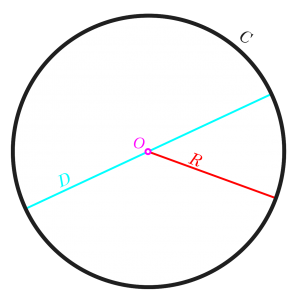The image above is a circle.

To compute the area of a circle, one essential parameter is needed and this parameter is the radius of a circle (r). You can also use the diameter of a circle to compute the area of a circle (d).

The formula for calculating the area of a circle is:

A = πr2

Where:

A = Area of a circle
r = Radius of a circle

Let’s solve an example:
Find the area of a circle where the radius of a circle is 6 cm.

This implies that;
r = Radius of a circle = 6 cm

A = πr2
A = 3.142 (6)2
A = 3.142 (36)
A = 113.10

Therefore, the area of a circle is 113.10 cm2

Calculating the Area of a Circle using the Diameter of a Circle.

The formula is A = πd2/4

Where;
A = Area of a circle
d = Diameter of a circle

Let’s solve an example:
Find the area of a circle where the diameter of the circle is 7 cm.

This implies that;
d = Diameter of a circle = 4 cm

A = πd2 / 4
A = 3.142 (4)2 / 4
A = 3.142 (16) / 4
A = 3.142 (4)
A = 12.57

Therefore, the area of a circle with diameter given is 12.57 cm2

## The Use of Calculators in Underwater Research | Water Quality IndexUnderwater research has provided guidance and opportunities for original researches to be conducted in the field of subtidal marine ecology. The research has been devoted particularly to the physiological process and limits of breathing gas under pressure, for aquanaut and astronaut training as well as for research on marine ecology.

Water quality index (WQI) is a means by which water quality data is summarized for reporting to the public in a consistent manner. It is similar to UV index or air quality index. Water quality index, as an area of activity under underwater research, is a 100-point scale that summarizes results from a total of nine different measurements when complete. These nice factors which includes:- dissolved oxygen, fecal coliform, PH, biochemical oxygen demand, temperature change, total phosphate, nitrates, turbidity and total solids, are chosen and some judged more important than the other, after which a weighted mean is used to combine the values.

The updated calculator used for this computation allows one to enter the longitude and latitude for the site under consideration or pick location from a Google earth map. The calculator completes the individual and group calculations of the aforementioned factors under consideration and permits one to generate a customized report.
The water quality index has a legend for grading range of values gotten at the final stage of each computation, which suggests the state of water falling between each range. The six widely used legends are excellent, very good, good, fair, marginal and poor.

## How to Calculate and Solve the Centre of Gravity of a Segment of a Sphere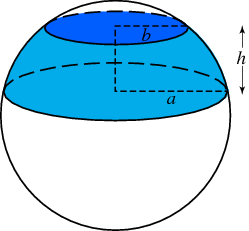The image above represents a segment of a sphere.
To compute the centre of gravity of a segment of a sphere requires two essential parameters. These parameters are the radius of the sphere and height of the segment of the sphere.

The formula for computing the centre of gravity of a sphere is:

C.G. = 3(2r – h)² / 4(3r – h)

Where:
C.G. = Centre of Gravity
r = Radius of the Sphere
h = Height of the Segment of the Sphere

Let’s solve an example
Find the centre of gravity of the segment of the sphere where the radius of the sphere is 10 m and the height of the segment of the sphere is 4 m.

This implies that:
r = Radius of the Sphere = 10
h = Height of the Segment of the Sphere = 4

C.G. = 3(2(10) – 4)² / 4(3(10) – 4)
C.G. = 3(20 – 4)² / 4(30 – 4)
C.G. = 3(16)² / 4(26)
C.G. = 3(256) / 104
C.G. = 768 / 104
C.G. = 7.38

Therefore, the centre of gravity of the segment of the sphere is 7.38.

Nickzom Calculator – The Calculator Encyclopedia is capable of calculating the centre of gravity of a segment of a sphere at a height, h at a distance from the centre of the sphere measured along the height.

## How to Calculate and Solve for the Centre of Gravity of a Cube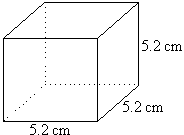The image above is a cube with a length of 5.2 cm.
To compute the centre of gravity of a cube, one essential parameter is needed and this parameter is the length of the cube (l).

The formula for calculating the centre of gravity of a cube is:

C.G. = 0.5(l)

Where:
l = Length of the Cube
C.G. = Centre of Gravity

Let’s solve an example:
Find the centre of gravity of a cube where the length of a side of the cube is 5.2 cm.

This implies that:
l = Length of the Cube = 5.2

## How to Calculate and Solve for the Centroid or Centre of Gravity of a HemisphereThe image above is a hemisphere with a radius of 5.
To compute the centroid or centre of gravity of a hemisphere. You need one essential parameter and this parameter is the radius of the hemisphere (r).

The formula for calculating the centroid or centre of gravity of a hemisphere is:

C.G. = 3r / 8

Where
r = Radius of the hemisphere

As always let us try and solve an example:
Find the centroid or centre of gravity of a hemisphere where the radius is 5 cm.

From the formula this implies that:
r = Radius of the hemisphere = 5

C.G. = 3(5) / 8
C.G. = 15 / 8
C.G. = 1.875

Therefore, the centroid or centre of gravity of the hemisphere is 1.875.

Nickzom Calculator – The Calculator Encyclopedia is capable of calculating the centre of gravity of a hemisphere at a distance from its base measured along the vertical radius.

To get the answer and workings of the center of gravity or centroid of a hemisphere using the Nickzom Calculator – The Calculator Encyclopedia. First, you need to obtain the app.

You can get this app via any of these means:

## Nickzom Calculator Calculates the Centroid or Centre of Gravity of a Semicircle | Statics (Mechanics)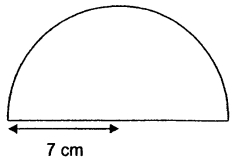The image above is a semicircle with a radius of 7 cm.
To compute the centroid or centre of gravity of a semicircle, you need one essential parameter and this parameter is the radius of the semicircle.

The formula for calculating the centroid or centre of gravity of a semicircle is:

C.G.= 4r /

Where:
r = Radius of the Semicircle
π = Mathematical Constant = 3.142 (approximately)

Let’s solve an example:
Find the centroid or centre of gravity of a semicircle where the radius is 7 cm.

From the example above,
r = radius of the semicircle = 7

C.G. = 4(7) /
C.G. = 28 / 9.4247
C.G. = 2.97

Therefore, the centroid or centre of gravity of the semicircle is 2.97.

Nickzom Calculator – The Calculator Encyclopedia is capable of calculating the centre of gravity of a semicircle at a distance from its base measured along the vertical radius.

To get the answer and workings of the center of gravity or centroid of a semicircle using the Nickzom Calculator – The Calculator Encyclopedia. First, you need to obtain the app.